# Which Bulb Glows Brighter When Connected in Series & Why?

We can connect the bulbs in the series or parallel according to the desired application. The lumen of the bulb depends on the current flowing through the bulb element.The heating effect depends on theand the resistance of the bulb. The heat produced by the bulb is proportional to the product of the square of the current and the resistance.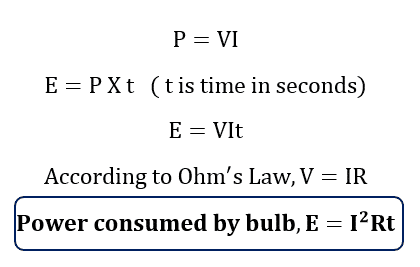Thus the heat produced depends on the current and the resistance of the bulb. By taking these two parameters into consideration we can find out the brightness of the bulbs when connected in the series or parallel. To understand, we will take two different cases of bulbs connected in the series and parallel combinations.

## Case 1: When identical rating bulbs are connected in the series

Let the voltage and power rating of the bulb is the same. The voltage, power of the bulbs are V and P respectively. The circuit diagram of identical bulbs connected in the series is as given below.

Let the voltage and power rating of the bulb is the same. The voltage, power of the bulbs are V and P respectively. The resistance of both the bulbs gets added and thus the current through the bulbs is half of its rated current. In this case, both the bulbs will glow with half of their rated intensity.

## Case 2- When identical rating bulbs are connected in the series and twice of                the bulb’s rated voltage is applied to a series circuitCurrent through series bulbs is;

I = 2V/(R+R) = 2V/2R = V/R

Thus, the current through each bulb is equal to bulb rated current, and both the bulb will glow with the same brightness.

This type of series lamp connection is used to measure 440 volts line to line voltage.Caution:

Both the bulbs should be of the same voltage 220 volts and the power of the bulb must be the same. In the case of different power ratings, the lower wattage bulb will explode due to more heating than its rated capacity and it may cause accidents.

## Case 3- When nonidentical rating bulbs are connected in the seriesResistance of Bulb 1 is ;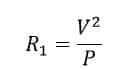Resistance of Bulb 2From above, it is clear that R2 >R1

The current flow through both the series-connected bulbs is the same.

The heat generated in bulb-1  is;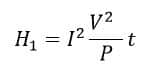The heat generated in bulb-2 is;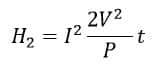From above H2>H1, therefore the bulb 2 will glow brighter than bulb 1.

### General Rule

When we connect two bulbs or more than two bulbs  in the series, the bulb which has lower power rating have more resistance and it will glow brighter.

Example:

Two bulbs of power rating 50- watt and 100-watt, 220 volts are connected in the series and fed 220 volts. Which bulb will glow brighter?

Resistance of 50-Watt bulbResistance of 100-watt bulb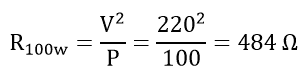The total resistance of the series circuit (R)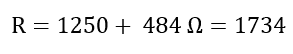The total current in the circuit (I)The current flowing through both series-connected bulbs will be the same.

The power dissipated by a 50-watt bulb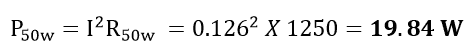The power dissipated by a 50-watt bulb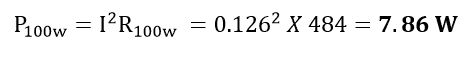From above, it is clear that a 50 watts bulb draws more power than a 100-watt bulb.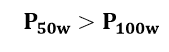Therefore, a 50-watt bulb will glow brighter than a 100-watt bulb when connected in the series.

Related Articles